Trigonometric Functions and Their Graphs:
The Co-Functions
(page 3 of 3)

Sections: The sine and cosine, The tangent, The co-functions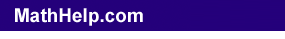What about the co-functions, the secant, the cosecant, and the cotangent?

The cosecant is the reciprocal of the sine. Wherever the sine is zero, the cosecant will be undefined, so there will be a vertical asymptote. Wherever the sine reaches its maximum value of 1, the cosecant will reach its minimum value of 1; wherever the sine reaches its minimum value of –1, the cosecant will reach its maximum value of –1. Wherever the sine is positive but less than 1, the cosecant will be positive but greater than 1; wherever the sine is negative but greater than –1, the cosecant will be negative but less than –1.

 So I'll lightly draw the sine wave... Copyright © Elizabeth Stapel 2010-2011 All Rights Reserved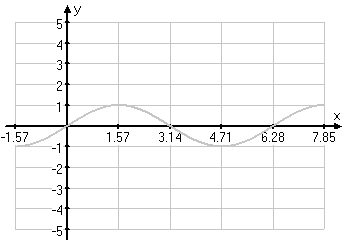...I'll draw vertical asymptotes through its zeroes and note the min/max points...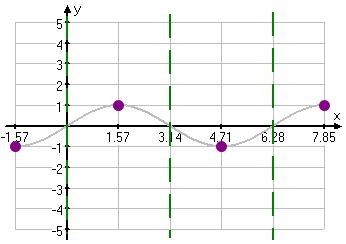...and then I'll fill in the graph.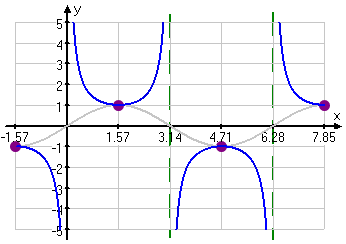The Cosecant Graph

By using the same reasoning with the cosine wave, I can create the secant graph: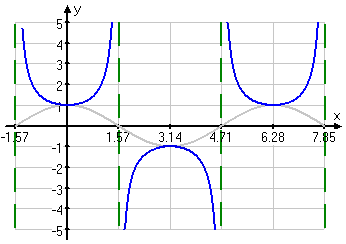The Secant Graph

The secant and cosecant have periods of length , and we don't consider amplitude for these curves.

Content Continues Below

The cotangent is the reciprocal of the tangent. Wherever the tangent is zero, the cotangent will have a vertical asymptote; wherever the tangent has a vertical asymptote, the cotangent will have a zero. And the signs on each interval will be the same. So the cotangent graph looks like this: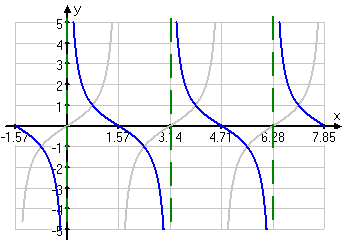The Cotangent Graph

The cotangent has a period of π, and we don't bother with the amplitude.

When you need to do the graphs, you may be tempted to try to compute a lot of plot points. But all you really need to know is where the graph is zero, where it's equal to 1, and / or where it has a vertical asymptote. If you know the behavior of the function at zero, π/2, π, 3π/2, and , then you can fill in the rest. That's really all you "need".

<< Previous  Top  |  1 | 2 | 3  |  Return to Index

 Cite this article as: Stapel, Elizabeth. "Trigonometric Functions and Their Graphs: Co-functions." Purplemath. Available from     https://www.purplemath.com/modules/triggrph3.htm. Accessed [Date] [Month] 2016

MathHelp.com Courses

Purplemath:

Study Skills Survey